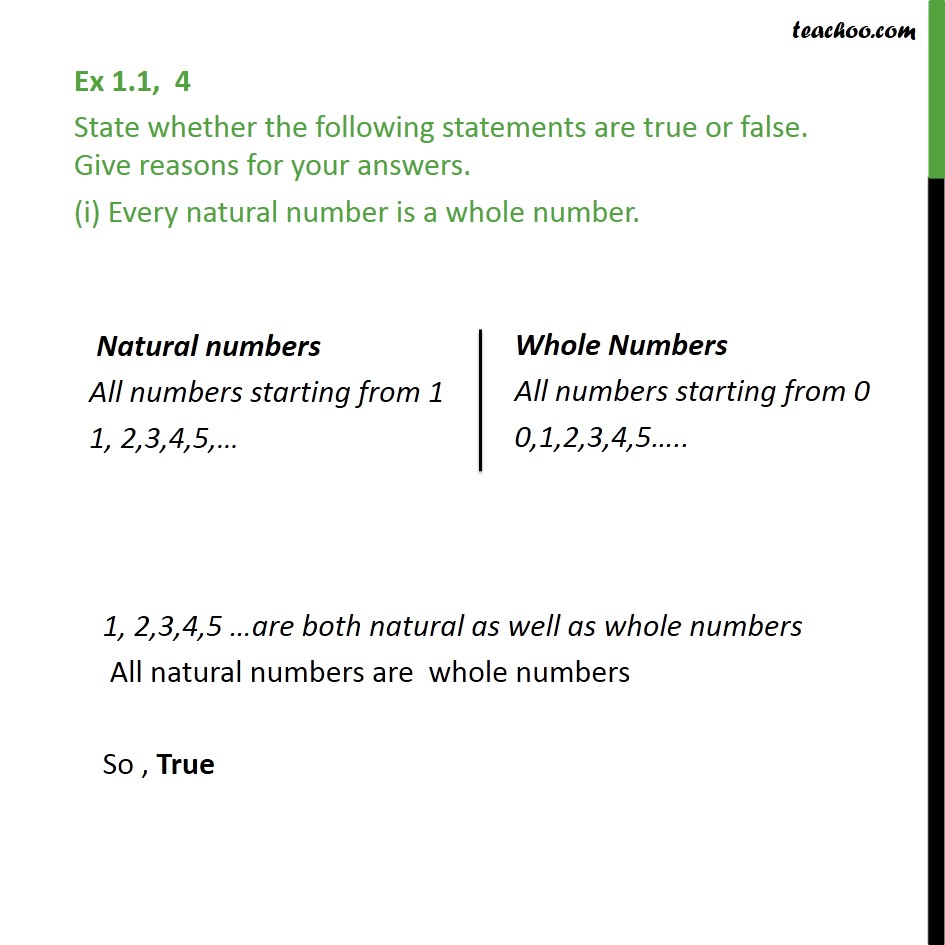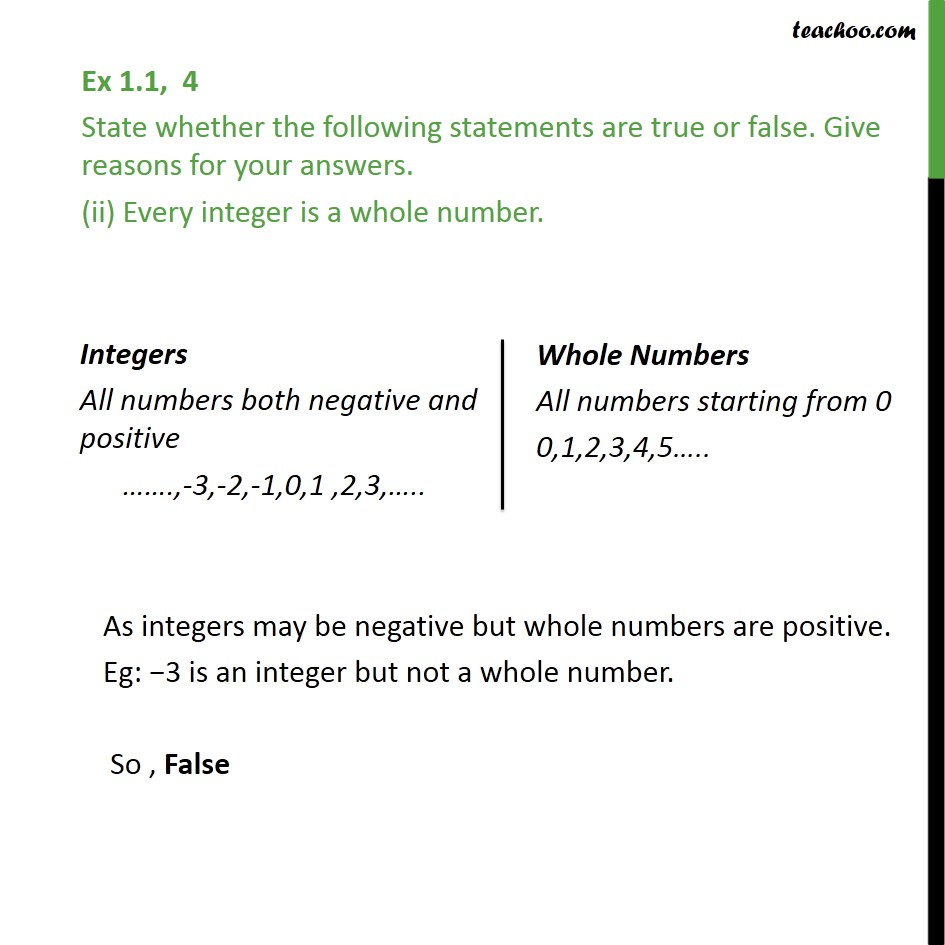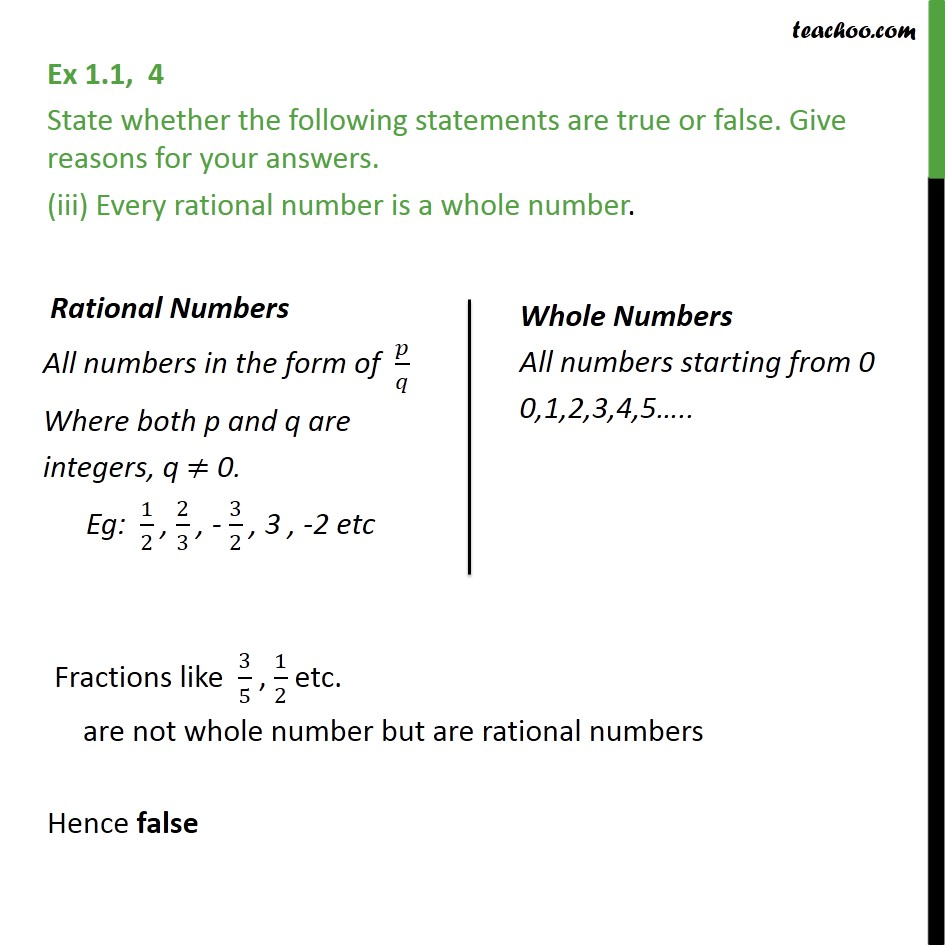1. Chapter 1 Class 9 Number Systems (Term 1)
2. Serial order wise
3. Ex 1.1

Transcript

Ex 1.1, 4 State whether the following statements are true or false. Give reasons for your answers. (i) Every natural number is a whole number. 1, 2,3,4,5 are both natural as well as whole numbers All natural numbers are whole numbers So , True Ex 1.1, 4 State whether the following statements are true or false. Give reasons for your answers. (ii) Every integer is a whole number. As integers may be negative but whole numbers are positive. Eg: 3 is an integer but not a whole number. So , False Ex 1.1, 4 State whether the following statements are true or false. Give reasons for your answers. (iii) Every rational number is a whole number. Fractions like 3/5 , 1/2 etc. are not whole number but are rational numbers Hence false

Ex 1.1